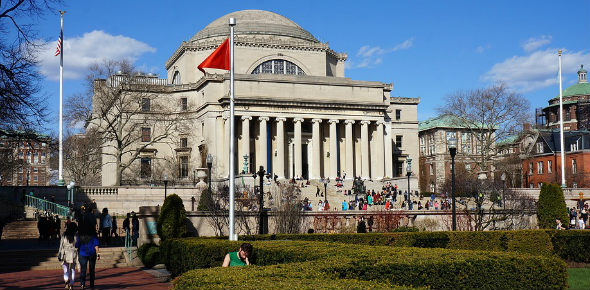# Dimensional Analysis And Similitude

14 Questions | Total Attempts: 660SettingsThis is prepared for SASTRA University students by Dr. T. R. Neelakantan, Prof. , School of Civil Engineering, SASTRA University, Thanjavur, Tamilnadu, India.

• 1.
1. Match List I with List II and select the correct answer using the codes given below the lists:List I(Fluid problems)Flow over a spillway damFlow through a butterfly valveRise of moisture in the steam of a plantWater hammer in a penstockList II(Model laws)Euler NumberFroude NumberMach NumberReynolds NumberWeber Number
• A.

A-2 B-4 C-5 D-3

• B.

A-4 B-2 C-3 D-1

• C.

A-2 B-3 C-1 D-4

• D.

A-5 B-1 C-4 D-2

• 2.
Match List I with List II and select the correct answer using the codes given below the lists: List I (Flow problem under study) Rise of gas bubbles in liquid Flow of gas in a pipe Flow over a spillway dam Flight of supersonic jet List II (Model laws) Euler Number Froude Number Mach Number Reynolds Number Weber Number
• A.

A-3 B-5 C-4 D-1

• B.

A-3 B-4 C-2 D-1

• C.

A-5 B-2 C-4 D-3

• D.

A-5 B-4 C-2 D-3

• 3.
Both Reynolds and Froude numbers assume significance in one of the following examples:
• A.

Motion of a submarine at large depths

• B.

Motion of ship in rough seas

• C.

Cruising of a missile in air

• D.

Flow over spillways

• 4.
Dynamic similarity is said to exist between the flow over two geometrically similar bodies when
• A.

Geometrical similarity exists between the two flows

• B.

Velocities at corresponding points are the same

• C.

The flow fields have geometrical similarity and force field similarity

• D.

The forces acting on the bodies are equal.

• 5.
If two geometrically similar models having a scale ratio of Lr are operated in a given laboratory at the same Froude number, then all the corresponding accelerations will be in the ratio of
• A.

Lr^1.5

• B.

Lr

• C.

Lr^0.5

• D.

1

• 6.
Match List I with List II and select the correct answer using the codes given below the lists: List I (Parameters) Inertial forces and gravitational force Inertial forces and elastic forces Inertial forces and surface tension Inertial forces and viscous force List II (Number) Reynolds Number Weber Number Froude Number Mach Number
• A.

A-2 B-3 C-4 D-1

• B.

A-1 B-4 C-2 D-3

• C.

A-3 B-4 C-2 D-1

• D.

A-3 B-2 C-4 D-1

• 7.
A river model is constructed to a horizontal scale of 1:1000 and a vertical scale of 1:100.  If the model discharge were 0.1m3/s, then the discharge in the river (in m3/s) would be
• A.

1,000

• B.

10,000

• C.

100,000

• D.

1000,000

• 8.
Match List I with List II and select the correct answer using the codes given below the lists: List I (Non-dimensional numbers) Mach number Thoma number Reynolds number Weber number List II (Application) 1. Waves in an ocean2. Launching of rockets3. Cavitation phenomenon4. Capillary flow in soil 5. Motion of a submarine
• A.

A-1 B-3 C-5 D-2

• B.

A-2 B-3 C-5 D-4

• C.

A-3 B-2 C-4 D-1

• D.

A-2 B-1 C-3 D-4

• 9.
A spillway model constructed to a scale of 1:4 gives a discharge of 5 m3/s.   The discharge from the prototype would be
• A.

160 m3/s

• B.

640 m3/s

• C.

10 m3/s

• D.

20 m3/s

• 10.
A river model is made to a length scale ratio of 1/100 and depth scale ratio of 1/16.  A peak discharge of 25,600 m3/s in the river will be simulated in the model with a discharge of
• A.

10 cumec

• B.

4 cumec

• C.

1.6 cumec

• D.

0.64 cumec

• 11.
If the physical quantities involved in a fluid flow phenomenon are discharge Q, diameter D, acceleration due to gravity g, dynamic viscosity μ and density ρ then the number of π parameters needed to express the function F (Q, D, g, m, ρ) = 0 are
• A.

2

• B.

3

• C.

4

• D.

5

• 12.
In a 1: 100 scale model of a harbour, the time which will correspond to the prototype tidal period of 12 hours will be
• A.

0.12 hour

• B.

1.2 hours

• C.

12 hours

• D.

120 hours

• 13.
Apart form inertia force, which of the following forces is most important in motion of submarines under water?
• A.

Viscous force

• B.

Gravity force

• C.

Compressive force

• D.

Surface tension force

• 14.
A model of reservoir is emptied in 10 minutes.  If the model scale is 1:25, the time taken by the prototype to empty itself, would be
• A.

250 minutes

• B.

50 minutes

• C.

6250 minutes

• D.

2 minutes

Related TopicsBack to top